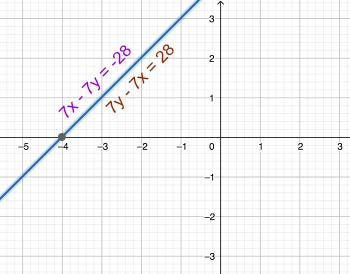# Solve the system of equations by graphing. Then classify the system as consistent or inconsistent...

## Question:

Solve the system of equations by graphing. Then classify the system as consistent or inconsistent and as dependent or independent.

{eq}7x - 7y = -28 ; {/eq} {eq}7y - 7x = 28. {/eq}

## Solving Linear Systems of Equations by Graphing:

In mathematics, we solve a linear system of equations by graphing the lines in the system on the same graph, and identifying their intersection point(s). If they intersect exactly once, then the system has exactly one solution at that intersection point, if they are the same line, meaning they intersect everywhere, then the system has infinitely many solutions, and if they are parallel and not the same line, then the system has no solutions.

The system of equations given has infinitely many solutions, so it is a consistent and dependent system. We have the following rules for classifying linear systems of equations.

• If a system of equations has a solution, then the system is consistent.
• If a system of equations has exactly one solution, then it is consistent and independent.
• If a system of equations has infinitely many solutions, then it is consistent and dependent.
• If a system of equations has no solutions, then it is inconsistent.

To determine the solution and classification of our system, we plot both of the equations in the system on a graph, and determine the solutions from that graph as the intersection point(s) of the lines. We then use that solution to classify the system.We see that the graph of {eq}7x-7y=-28 {/eq} and {eq}7y-7x=28 {/eq} are the same line. Therefore, they intersect everywhere and the system has infinitely many solutions. Since the system has infinitely many solutions, we have that it is a consistent and dependent system.